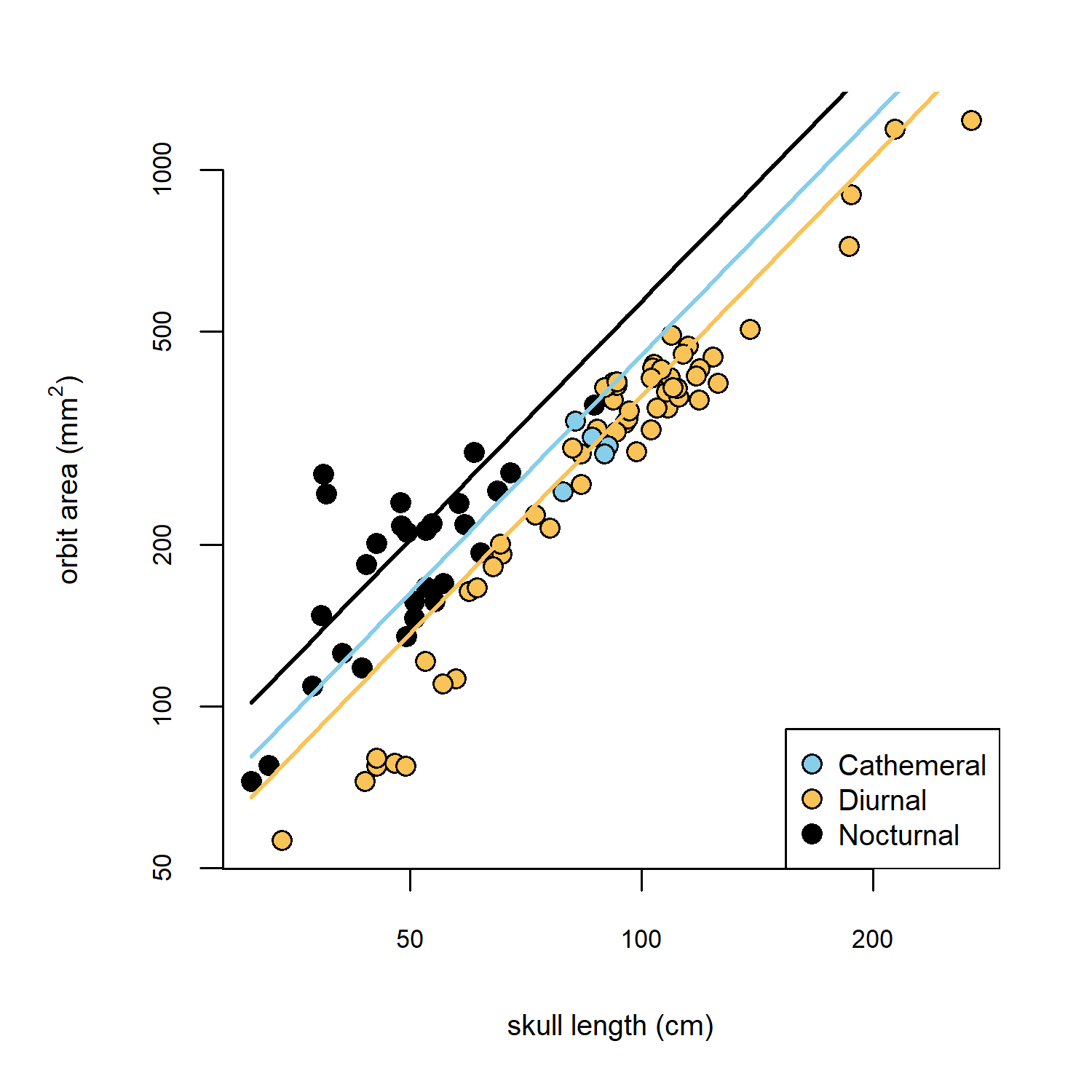### 1. Coding of Ateles (spider monkeys) in primate dataset

An astute reader of our book, and follower of my blog, recently pointed out that Ateles (the spider monkeys) were incorrectly coded as cathemeral in our dataset for phylogenetic ANCOVA of this chapter, when they should’ve properly been coded as unambiguously diurnal.

Honestly, I cannot account for where this error originates. Fortunately, it does not qualitatively affect any of the analyses given in the chapter. Nonetheless, the results are quantitatively affected (in the sense that the values of coefficients and test statistics change slightly) when this error is corrected, so I thought it would be worthwhile to post the corrected results here. To ensure that readers of the book obtain quantitatively equivalent results to those of the published version, we will not replace the posted data file; however, I have now included the corrected primates dataset of the book chapter with phytools (>= 1.7-8), so readers with that version of the package can follow along below.

## load nlme
library(nlme)
library(phytools)
## check package version
packageVersion("phytools")
##  '1.7.8'
## load primate dataset and tree
data(primate.tree)
data(primate.data)
head(primate.data[,5:6],10)
##                             Activity_pattern Activity_pattern_code
## Allenopithecus_nigroviridis          Diurnal                     0
## Alouatta_palliata                    Diurnal                     0
## Alouatta_seniculus                   Diurnal                     0
## Aotus_trivirgatus                  Nocturnal                     2
## Arctocebus_aureus                  Nocturnal                     2
## Arctocebus_calabarensis            Nocturnal                     2
## Ateles_fusciceps                     Diurnal                     0
## Ateles_geoffroyi                     Diurnal                     0
## Ateles_paniscus                      Diurnal                     0
## Avahi_laniger                      Nocturnal                     2

The diel activity pattern of all Ateles species can now be seen to be correctly coded as Diurnal rather than Cathemeral.

Now let’s re-run our analyses.

## create correlation structure
spp<-rownames(primate.data)
corBM<-corBrownian(phy=primate.tree,form=~spp)
## fit ANCOVA model
primate.ancova<-gls(log(Orbit_area)~log(Skull_length)+
Activity_pattern,data=primate.data,
correlation=corBM)
anova(primate.ancova)
## Denom. DF: 86
##                   numDF   F-value p-value
## (Intercept)           1 2045.7504  <.0001
## log(Skull_length)     1  382.0018  <.0001
## Activity_pattern      2    9.8960   1e-04

The F-values and p-values of our fitted model coefficients change slightly, but not in a substantive way compared to the analysis that was published in the book.

## set the margins of our plot using par
par(mar=c(5.1,5.1,2.1,2.1))
## set the point colors for the different levels
## of our factor
pt.cols<-setNames(c("#87CEEB","#FAC358","black"),
levels(primate.data$Activity_pattern)) ## plot the data plot(Orbit_area~Skull_length,data=primate.data,pch=21, bg=pt.cols[primate.data$Activity_pattern],
log="xy",bty="n",xlab="skull length (cm)",
ylab=expression(paste("orbit area (",mm^2,")")),
cex=1.2,cex.axis=0.7,cex.lab=0.8)
legend("bottomright",names(pt.cols),pch=21,pt.cex=1.2,
pt.bg=pt.cols,cex=0.8)
## create a common set of x values to plot our
## different lines for each level of the factor
xx<-seq(min(primate.data$Skull_length), max(primate.data$Skull_length),length.out=100)
## add lines for each level of the factor
lines(xx,exp(predict(primate.ancova,
newdata=data.frame(Skull_length=xx,
Activity_pattern=as.factor(rep("Cathemeral",100))))),
lwd=2,col=pt.cols["Cathemeral"])
lines(xx,exp(predict(primate.ancova,
newdata=data.frame(Skull_length=xx,
Activity_pattern=as.factor(rep("Diurnal",100))))),
lwd=2,col=pt.cols["Diurnal"])
lines(xx,exp(predict(primate.ancova,
newdata=data.frame(Skull_length=xx,
Activity_pattern=as.factor(rep("Nocturnal",100))))),
lwd=2,col=pt.cols["Nocturnal"])Figure 3.3 from the book, but updated based on the correction to diel activity pattern in spider monkeys (Ateles).